# 关于std::shared_ptr与std::enable_shared_from_this循环引用导致的问题

#include "stdafx.h"
#include <memory>

class A
{
public:
A()
{
m_i = 9;
}

~A()
{
m_i = 0;
}

public:
int     m_i;

};

int _tmain(int argc, _TCHAR* argv[])
{
{
std::shared_ptr<A> spa(new A());
}

return 0;
}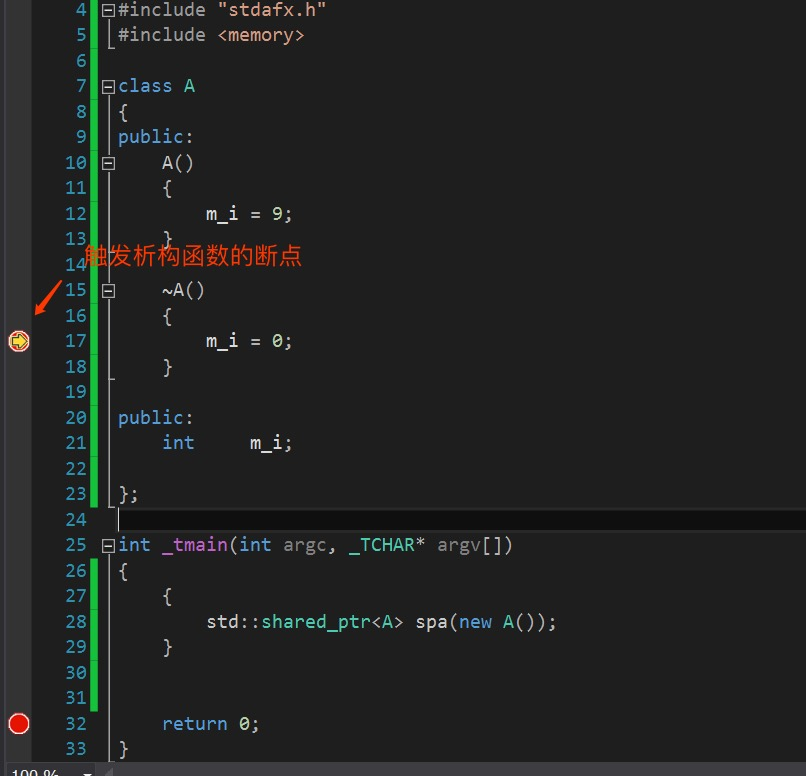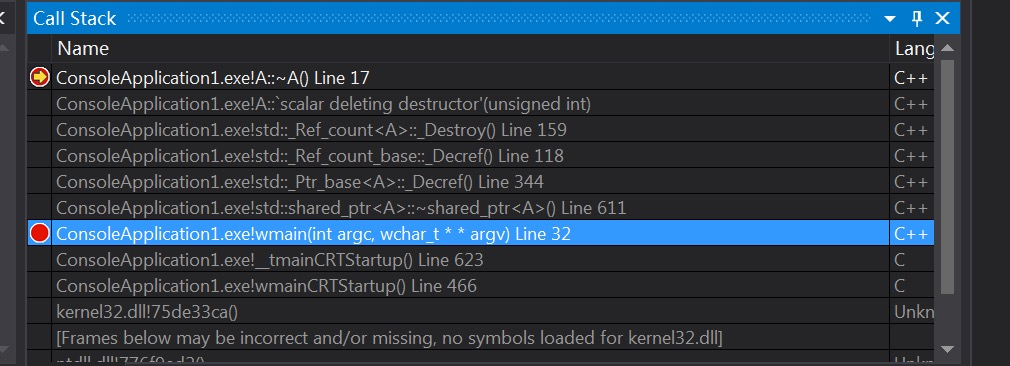#include "stdafx.h"
#include <memory>

class A : public std::enable_shared_from_this<A>
{
public:
A()
{
m_i = 9;
//注意:
//比较好的做法是在构造函数里面调用shared_from_this()给m_SelfPtr赋值
//但是很遗憾不能这么做,如果写在构造函数里面程序会直接崩溃
}

~A()
{
m_i = 0;
}

void func()
{
m_SelfPtr = shared_from_this();
}

public:
int                 m_i;
std::shared_ptr<A>  m_SelfPtr;

};

int _tmain(int argc, _TCHAR* argv[])
{
{
A a;
a.func();
}

return 0;
}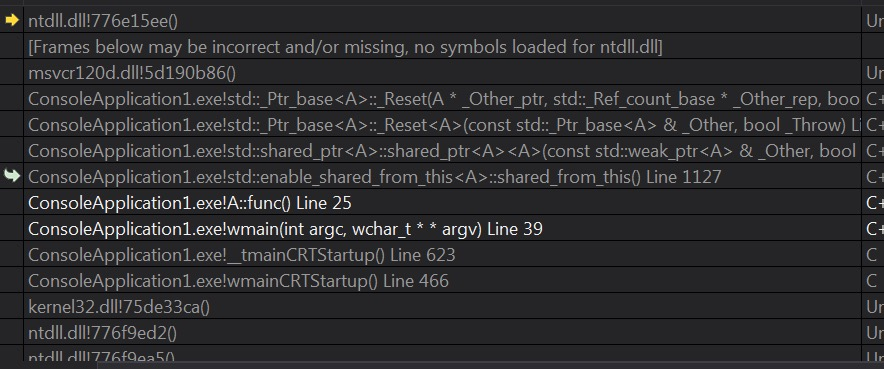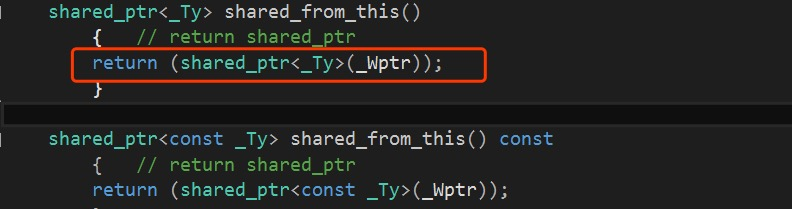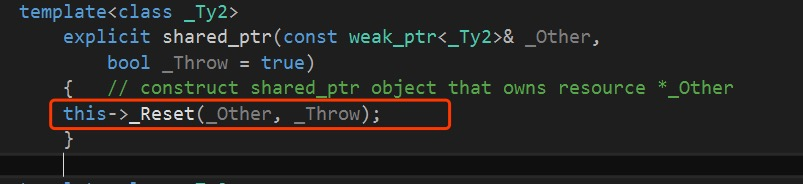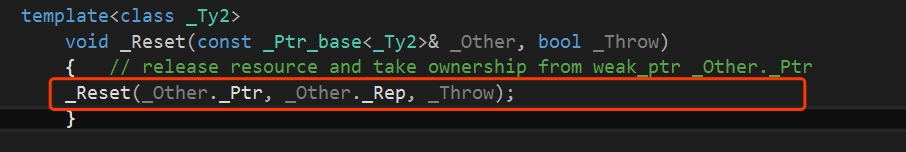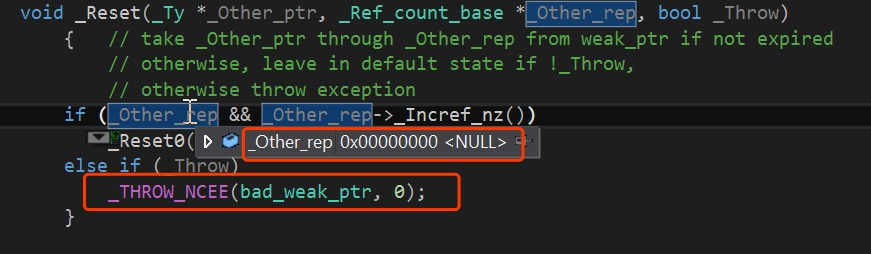private:
template<class _Ty1,
class _Ty2>
friend void _Do_enable(
_Ty1 *,
enable_shared_from_this<_Ty2>*,
_Ref_count_base *);

mutable weak_ptr<_Ty> _Wptr;



class A : public std::enable_shared_from_this<A>
{
public:
A()
{
m_i = 9;
//注意:
//比较好的做法是在构造函数里面调用shared_from_this()给m_SelfPtr赋值
//但是很遗憾不能这么做,如果写在构造函数里面程序会直接崩溃
}

~A()
{
m_i = 0;
}

void func()
{
m_SelfPtr = shared_from_this();
}

public:
int                 m_i;
std::shared_ptr<A>  m_SelfPtr;

};

int _tmain(int argc, _TCHAR* argv[])
{
{
std::shared_ptr<A> spa(new A());
spa->func();
}

return 0;
}

#include "stdafx.h"
#include <memory>

class A : public std::enable_shared_from_this<A>
{
public:
A()
{
m_i = 9;
//注意:
//比较好的做法是在构造函数里面调用shared_from_this()给m_SelfPtr赋值
//但是很遗憾不能这么做,如果写在构造函数里面程序会直接崩溃
}

~A()
{
m_i = 0;
}

void func()
{
m_SelfPtr = shared_from_this();
}

public:
int                 m_i;
std::shared_ptr<A>  m_SelfPtr;

};

int _tmain(int argc, _TCHAR* argv[])
{
std::weak_ptr<A> spwa;
{
std::shared_ptr<A> spa(new A());
spa->func();
spwa = spa;
}

printf("spwa usecount: %d\n", spwa.use_count());

return 0;
}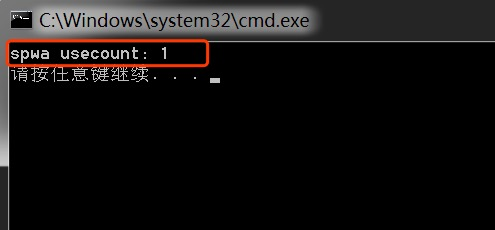#include "stdafx.h"
#include <memory>

class A : public std::enable_shared_from_this<A>
{
public:
A()
{
m_i = 9;
//注意:
//比较好的做法是在构造函数里面调用shared_from_this()给m_SelfPtr赋值
//但是很遗憾不能这么做,如果写在构造函数里面程序会直接崩溃
}

~A()
{
m_i = 0;
}

void func()
{
m_SelfPtr = shared_from_this();
}

void release()
{
m_SelfPtr.reset();
}

public:
int                 m_i;
std::shared_ptr<A>  m_SelfPtr;

};

int _tmain(int argc, _TCHAR* argv[])
{
std::weak_ptr<A> spwa;
{
std::shared_ptr<A> spa(new A());
spa->func();
spa->release();
spwa = spa;
}

printf("spwa usecount: %d\n", spwa.use_count());

return 0;
}


©️2019 CSDN 皮肤主题: 编程工作室 设计师: CSDN官方博客